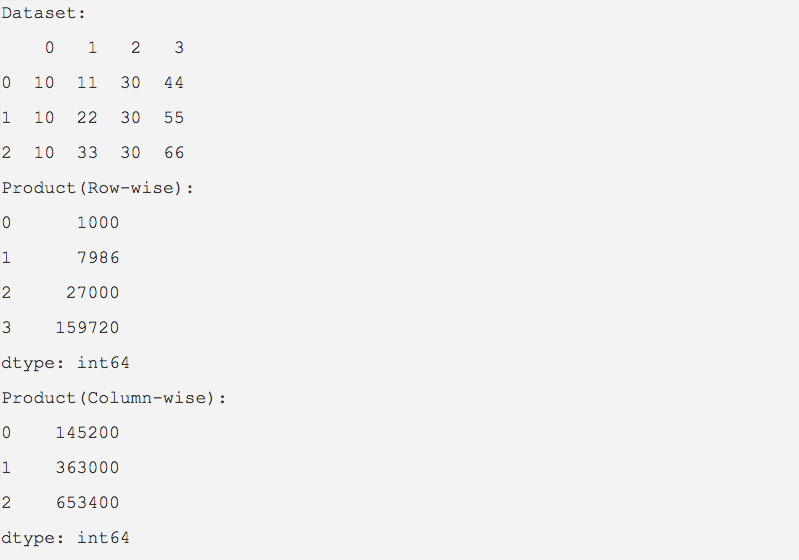# Product Of Values In A Pandas Dataframe

## Overview:

• The python library pandas provides essential data structures and methods to perform data analysis. The DataFrame class in pandas represents a 2 dimensional array.
• The rows and columns of a pandas DataFrame object can be multiplied and their product is returned as a pandas series using the prod() method .
• By default, the value for the axis parameter is 0, which calculates the product of columns.
• To have the data values of rows multiplied, use axis=1.

## Example:

 import pandas as pd   dataValue = [(10,11,30,44),              (10,22,30,55),              (10,33,30,66)] dataFrame   = pd.DataFrame(data=dataValue); print("Dataset:"); print(dataFrame);   product     = dataFrame.prod(); print("Product(Row-wise):"); print(product);   product     = dataFrame.prod(axis=1); print("Product(Column-wise):"); print(product);

## Output: